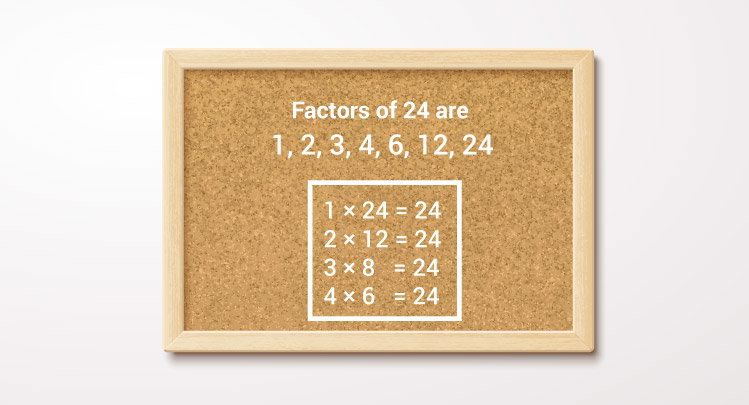# C Program to Display Factors of a Number

Example to find all factors of an integer (entered by the user) using for loop and if statement.To understand this example, you should have the knowledge of following C programming topics:

This program takes a positive integer from the user and displays all the positive factors of that number.

## Example: Factors of a Positive Integer

``````#include <stdio.h>
int main()
{
int number, i;

printf("Enter a positive integer: ");
scanf("%d",&number);

printf("Factors of %d are: ", number);
for(i=1; i <= number; ++i)
{
if (number%i == 0)
{
printf("%d ",i);
}
}

return 0;
}
``````

Output

```Enter a positive integer: 60
Factors of 60 are: 1 2 3 4 5 6 10 12 15 20 30 60
```

In the program, a positive integer entered by the user is stored in variable number.

The `for` loop is iterated until `i <= number` is false. In each iteration, whether number is exactly divisible by i is checked (condition for i to be the factor of number) and the value of i is incremented by 1.